﻿ 一种基于深度神经网络的译码器抽象方法 A Decoder Abstraction Method Based on Deep Neural Network

Hans Journal of Wireless Communications
Vol. 09  No. 03 ( 2019 ), Article ID: 30744 , 7 pages
10.12677/HJWC.2019.93013

A Decoder Abstraction Method Based on Deep Neural Network

Jinghui Xu, Yuehong Gao, Hongwen Yang

Department of Communication Engineering, School of Information and Communication Engineering, Beijing University of Posts and Telecommunications, BeijingReceived: May 22nd, 2019; accepted: Jun. 6th, 2019; published: Jun. 13th, 2019ABSTRACT

In the simulation of communication system, it is important to simulate the link decoding result accurately. This paper proposes a decoder abstraction method based on deep neural network (DNN), which extracts three features from the soft input of the decoder and uses the neural network model to predict the decoding success. Simulation results show that the method proposed in this paper has better prediction accuracy than traditional methods such as EESM.

Keywords:Deep Neural Network, Decoder Abstraction, Feature Extraction1. 引言

2. 系统模型

${y}_{k}={h}_{k}{s}_{k}+\sqrt{{P}_{I}}{g}_{k}{\stackrel{˜}{s}}_{k}+{z}_{k}$ (1)

${\gamma }_{k}=\frac{{|{h}_{k}|}^{2}}{{P}_{I}{|{g}_{k}|}^{2}+{\sigma }^{2}}$ (2)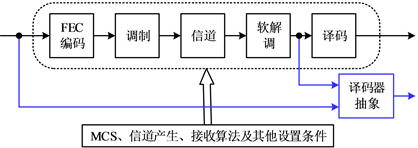Figure 1. Decoder abstraction

${\gamma }_{eff}=-\beta \mathrm{ln}\left(\frac{1}{N}\underset{k=1}{\overset{N}{\sum }}{e}^{-\frac{{\gamma }_{k}}{\beta }}\right)$ (3)

3. 基于DNN的译码器抽象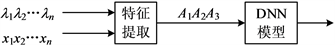Figure 2. The decoder abstraction unit in Figure 1

${A}_{1}=\frac{1}{2}\underset{i=1}{\overset{n}{\sum }}\left[1-\mathrm{sgn}\left({x}_{i}{\lambda }_{i}\right)\right]$ (4)

${A}_{2}=\frac{\underset{i=1}{\overset{n}{\sum }}{x}_{i}{\lambda }_{i}}{\sqrt{n\underset{i=1}{\overset{n}{\sum }}{\lambda }_{i}^{2}}}$ (5)

${A}_{3}=\underset{i=1}{\overset{n}{\sum }}\mathrm{ln}\left(1+{e}^{-{x}_{i}{\lambda }_{i}}\right)$ (6)

${p}_{i}=\frac{1}{1+{e}^{-{x}_{i}{\lambda }_{i}}}$ (7)

$\mathrm{Re}LU\left(x\right)=\left\{\begin{array}{cc}x,& x>0\\ 0,& x\le 0\end{array}$ (8)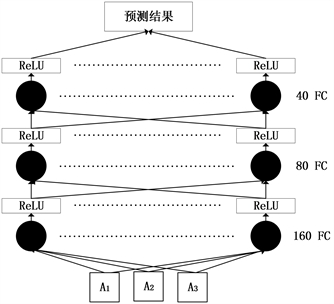Figure 3. DNN model

ReLU激活函数在DNN系统中引入了非线性因素。相比于其他激活函数，例如tanh和sigmoid，ReLU激活函数在处理梯度消失问题上的能力很强，这一能力也使得其训练速度更快  。虽然使用ReLU激活函数其节点对于梯度变化较为敏感，容易在收到较大梯度的冲击后进入非激活状态，但对于本文所研究的问题来说，由于系统规模较小，可以通过把学习速率控制在一个较小的数值，来保证其稳定性。

4. 仿真结果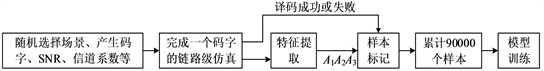(a) 所提方法的模型训练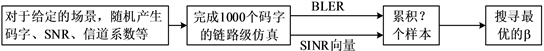(b) EESM参数优化

Figure 4. Model training and parameter optimization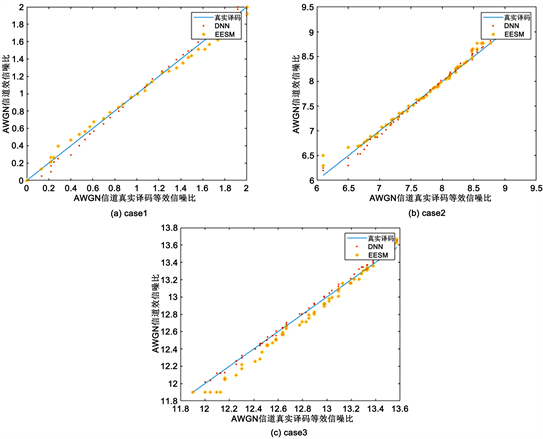Figure 5. The equivalent SNR under three scenarios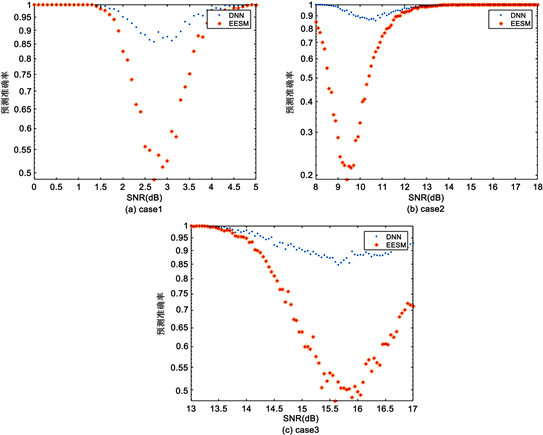Figure 6. Prediction accuracy of decoding results in three scenarios

5. 总结

A Decoder Abstraction Method Based on Deep Neural Network[J]. 无线通信, 2019, 09(03): 105-111. https://doi.org/10.12677/HJWC.2019.93013

1. 1. 陈伟, 孙引, 李云洲, 等. 基于Matlab的LTE系统级仿真平台的建立[J]. 通信技术, 2010, 43(5): 170-172.

2. 2. Capozzi, F., Piro, G., Grieco, L.A., et al. (2012) A System-Level Simulation Framework for LTE Femtocells. 5th International ICST Conference on Simulation Tools and Techniques, Desenzano del Garda, 19-23 March 2012, 211-213.
https://doi.org/10.4108/icst.simutools.2012.247767

3. 3. Ikuno, J.C., Wrulich, M. and Rupp, M. (2010) System Level Simulation of LTE Networks. 71st Vehicular Technology Conference, Taipei, 16-19 May 2010, 1-5.
https://doi.org/10.1109/VETECS.2010.5494007

4. 4. Lei, H., Zhang, L., Zhang, X., et al. (2007) System Level Evaluation of 3G Long Term Evolution. 18th International Symposium on Personal, Indoor and Mobile Radio Communications, Athens, 3-7 September 2007, 1-5.
https://doi.org/10.1109/PIMRC.2007.4394433

5. 5. Li, X., Fang, Q. and Shi, L. (2011) A Effective SINR Link to System Mapping Method for CQI Feedback in TD-LTE System. 2th International Conference on Computing, Control and Industrial Engi-neering, Wuhan, 20-21 August 2011, 208-211.
https://doi.org/10.1109/CCIENG.2011.6008103

6. 6. Hanzaz, Z. and Schotten, H.D. (2013) Impact of L2S Interface on System Level Evaluation for LTE System. 11th Malaysia International Conference on Communications, Kuala Lumpur, 26-28 November 2013, 456-461.
https://doi.org/10.1109/MICC.2013.6805873

7. 7. Gaveau, J., Martret, C.L. and Assaad, M. (2017) Grouping of Subcarriers and Effective SNR Statistics in Wideband OFDM Systems Using EESM. 13th IEEE International Conference on Wireless & Mobile Computing, Networking and Communications, Rome, 9-11 October 2017, 1-7.
https://doi.org/10.1109/WiMOB.2017.8115800

8. 8. 娄文科, 林辉, 曾志民. 基于互信息域的L2S接口方法研究[J]. 无线电通信技术, 2006, 32(5): 13-15.

9. 9. Aguilar, F.L., Cidre, G.R., López, J.M., et al. (2010) Mutual Information Effective SNR Mapping Algorithm for Fast Link Adaptation Model in 802.16e. In: Chatzimisios, P., et al., Eds., Mobile Lightweight Wireless Systems, Springer, Berlin Heidelberg, 356-367.
https://doi.org/10.1007/978-3-642-16644-0_31

10. 10. Li, Y., Long, H., Yang, H., et al. (2017) Coexistence of LTE and WLAN Systems and Improvement of L2S Interface. IEEE/CIC International Conference on Communications in China, Qingdao, 22-24 October 2017, 1-6.
https://doi.org/10.1109/ICCChina.2017.8330441

11. 11. Nie, W. and Yang, H. (2010) A New Link Level to System Level Simu-lation Interface Method. International Conference on Audio Language & Image Processing, Shanghai, 23-25 November 2010, 1311-1314.
https://doi.org/10.1109/ICALIP.2010.5685070

12. 12. Khan, A., Ullah, I. and Khattak, S. (2017) Link to System Interfacing for Multiple Input and Multiple Output Wireless System Using Maximum Likelihood Receiver. IET Communications, 11, 1346-1351.
https://doi.org/10.1049/iet-com.2016.0019

13. 13. Chu, E., Jang, H.J. and Jung, B.C. (2018) Machine Learning Based Link-to-System Mapping for System-Level Simulation of Cellular Networks. 10th International Conference on Ubiquitous and Future Networks, Prague, 3-6 July 2018, 503-506.
https://doi.org/10.1109/ICUFN.2018.8436754

14. 14. Hu, X.Y., Eleftheriou, E., Arnold, D.M., et al. (2001) Efficient Implementations of the Sum-Product Algorithm for Decoding LDPC Codes. 2nd IEEE Global Telecommunications Conference, Vol. 2, San Antonio, 25-29 November 2001, 1036.

15. 15. 何燕锋, 杨鸿文, 郭文彬. 高阶调制的软输出算法比较[J]. 北京邮电大学学报, 2003, 26(1): 82-85.

16. 16. Dahl, G.E., Sainath, T.N. and Hinton, G.E. (2013) Improving Deep Neural Networks for LVCSR Using Rectified Linear Units and Dropout. Acoustics, Speech and Signal Processing, Vancouver, 26-30 May 2013, 8609-8613.